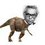# Simple concurrency

In $\Delta ABC$ , let $D$ be any point on $\overline{BC}$.Let $\overline{DF} \ , \ \overline{DE}$ be bisectors of $\angle ADB \ , \ \angle ADC$ respectively with $E \in \overline{AC} \ , \ F \in \overline{AB}$ . Prove that $\overline{AD} \ , \ \overline{BE} \ , \ \overline{CF}$ are concurrent.

###### Posed this while playing with a theorem ;)Note by Nihar Mahajan
5 years, 10 months ago

This discussion board is a place to discuss our Daily Challenges and the math and science related to those challenges. Explanations are more than just a solution — they should explain the steps and thinking strategies that you used to obtain the solution. Comments should further the discussion of math and science.

When posting on Brilliant:

• Use the emojis to react to an explanation, whether you're congratulating a job well done , or just really confused .
• Ask specific questions about the challenge or the steps in somebody's explanation. Well-posed questions can add a lot to the discussion, but posting "I don't understand!" doesn't help anyone.
• Try to contribute something new to the discussion, whether it is an extension, generalization or other idea related to the challenge.

MarkdownAppears as
*italics* or _italics_ italics
**bold** or __bold__ bold
- bulleted- list
• bulleted
• list
1. numbered2. list
1. numbered
2. list
Note: you must add a full line of space before and after lists for them to show up correctly
paragraph 1paragraph 2

paragraph 1

paragraph 2

[example link](https://brilliant.org)example link
> This is a quote
This is a quote
    # I indented these lines
# 4 spaces, and now they show
# up as a code block.

print "hello world"
# I indented these lines
# 4 spaces, and now they show
# up as a code block.

print "hello world"
MathAppears as
Remember to wrap math in $$ ... $$ or $ ... $ to ensure proper formatting.
2 \times 3 $2 \times 3$
2^{34} $2^{34}$
a_{i-1} $a_{i-1}$
\frac{2}{3} $\frac{2}{3}$
\sqrt{2} $\sqrt{2}$
\sum_{i=1}^3 $\sum_{i=1}^3$
\sin \theta $\sin \theta$
\boxed{123} $\boxed{123}$

Sort by:

Angle bisector theorem and cevas

- 5 years, 10 months ago

Ah , I knew you would get it quickly. I used Ceva's instead of Menelaus. Nevermind Ceva's and Menelaus are brothers ;) :P

- 5 years, 10 months ago

I actually meant to type ceva...haven't used the two in a while so got them mixed up haha

- 5 years, 10 months ago

Lol , okay...

- 5 years, 10 months ago

Can you prove the following simple fact (I tried to devise a problem out of this configuration):

Suppose the circumcircle of $DEF$ meets $AD$ again at $X$. Prove that the reflection of $X$ over $EF$ lies on $BC$

- 5 years, 10 months ago

That was nice. Let $\overline{BC} \cap \odot DEF = \{X'\}$ and I claim that $X'$ is the reflection of $X$. To prove this its easy to see that $\Delta XFE \cong \Delta X'FE$ which makes $\Box XFX'E$ a kite. This implies $\overline{XX'} \perp \overline{FE}$ and $XK=KE$ provided that $\overline{FE} \cap \overline{XX'} = \{K\}$. Thus it is proved that $X'$ is the reflection of $X$ such that $X' \in \overline{BC}$

- 5 years, 10 months ago

Which theorem?

- 5 years, 10 months ago

I won't give away the name unless a solution is posted here ;)

- 5 years, 10 months ago

ceva theorm has something with concurrent....

- 5 years, 10 months ago

It does not have "something" , it is fully based on concurrency :)

- 5 years, 10 months ago

Now you can see that some people have already taken its name ;)

- 5 years, 10 months ago

we need to prove (BD/DC)(CE/AE)(AF/BF) = 1

- 5 years, 10 months ago

Where's the proof? You only stated what to prove.

- 5 years, 10 months ago

my geometry is weak...please show proof.

- 5 years, 10 months ago

Such an easy one. Use ceva's theorem and angle bisector property.

- 5 years, 10 months ago

Try proving it with another method.

- 5 years, 10 months ago

We can prove it by co-ordinate geometry but then it would be tedious.

- 5 years, 10 months ago

Nihar, I suppose u are an Indian. If u like geometry try solving INMO 1994 question number 5. It is in one of my notes(rather the only note). https://brilliant.org/discussions/thread/amazing-geometry-question/

- 5 years, 10 months ago